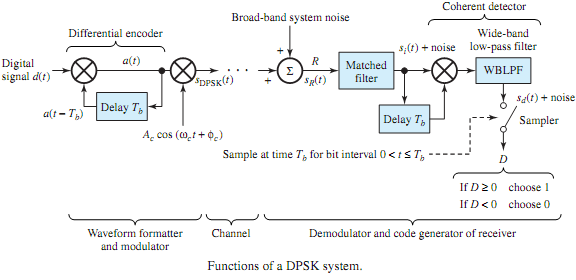## Illustrate differential phase-shift keying, Electrical Engineering

Assignment Help:

Q. Illustrate Differential phase-shift keying?

In order to eliminate the need of a local carrier, DPSK has been developed in which the receiver uses the received signal to act as its own carrier. Figure shows the functions of a DPSK system in which the leftmost product operation along with 1-bit delay is the differential encoder. The digital signal d(t) is a polar waveform of levels ±1, corresponding to binary digits 1 and 0, respectively. The output signal a(t) from the differential encoder PSK-modulates a carrier to produce the DPSK signal sDPSK(t). The product device followed by the wide-band low-pass filter acts as the coherent detector. The two inputs to the product device are obtained from the output of a filter matched to the input pulse in a single bit interval. Note that the 1-bit delayed input to the product device serves the purpose of the local oscillator for the coherent detector; that is to say, the DPSK waveform in a given bit interval serves as its own local-oscillator signal in the following bit interval.In Figure, si(t) is the signal component of the matched filter output, and sd(t)isthe signal component of the detector output. If the phases of both si(t) and si(t - Tb) are the same, sd(t) is then a positive voltage; if their phases differ by π radians, sd(t) will then be a  negative voltage. These voltages will have maximum amplitudes at the sample time at the end of the bit interval. Because the sign of the voltage at the sampler depends upon the phase relationship between si(t) and its delayed replica, and the sign of sd(t) is of the same form as d(t), the original digital bit sequence can be determined by sampling to decide the sign of the detector output. Figure illustrates an example sequence of message binary digits, modulator wave- forms in DPSK, and phase and polarity relationships as applied to DPSK message recovery.

#### Single phase full wave controlled rectifier , Single Phase Full Wave Contro...

Single Phase Full Wave Controlled rectifier The single  phase  half  wave controlled  rectifier  produce only one pulse  of load  current  during  one cycle  of supply voltage

#### Circuit realization of the srff, Q. You are to construct a modified truth t...

Q. You are to construct a modified truth table for the circuit realization of the SRFF shown in Figure. As indicated in the text, you guess an output and then go back ato check it

#### Explain the excess three codes - bcd to decimal conversion, Explain the Exc...

Explain the Excess three codes - BCD to Decimal Conversion The Excess-3 representation of a number is based on Binary Coded Decimal. It is formed by taking the number in Binary

#### Common-drain jfet amplifier, Q. Common-Drain JFET Amplifier? Figure (a)...

Q. Common-Drain JFET Amplifier? Figure (a) shows a CD JFET amplifier in which resistors R 1 , R 2 , and R SS are selected by the bias design, and capacitors CG and CS are chos

#### Module of the rated currents - transformer, Module of the rated currents - ...

Module of the rated currents - transformer: The transformer of the Face(Figure) 2 is a transformer of type Delta-Y (the neutral of Y is grounded). The rated output is of 1500

#### Find the ratio of the forward to the reverse current, Assume that an abrupt...

Assume that an abrupt Si p-n junction with area 10-4 cm2 has NA=10 17 /cm 3 and ND=10 17 /cm 3 is working at room temperature. It is given that μ n = 700 cm 2 /v-s, μ P =250 cm

#### Required conditions for connecting two transformers, Q. Required Conditions...

Q. Required Conditions for connecting two transformers in parallel? Ans: a) Voltage rating should be same b) Per unit impedance should be same c) Phase sequence should

#### Illustrate sources and loads, Q. Illustrate Sources and Loads? A source...

Q. Illustrate Sources and Loads? A source-load combination is represented in Figure. A node is a point at which two or more components or devices are connected together. A part

#### Illustrate the basic concept of machine cell design, a) Write short notes o...

a) Write short notes on part feeding devices and transfer mechanism. b) Explain with example automation for machining operation with relevant diagrams. c) discuss different p

#### Differentiate between an integrator and differentiator, Q . Differentiate b...

Q . Differentiate between an integrator and differentiator? Integrators 1 Integrators are circuits in which output voltage is proportional to the integral of the input.